International Journal of Statistical Distributions and Applications
Volume 1, Issue 1, September 2015, Pages: 12-18

Optimal Search and Rescue Model: Updating Probability Density Map of Debris Location by Bayesian Method

Department of Mathematics, Sichuan University, Chengdu, China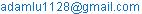(Lu Yadong)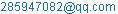(Zhou Ya)

Lu Yadong, Zhou Ya. Optimal Search and Rescue Model: Updating Probability Density Map of Debris Location by Bayesian Method. International Journal of Statistical Distributions and Applications. Vol. 1, No. 1, 2015, pp. 12-18. doi: 10.11648/j.ijsd.20150101.13

Abstract: Optimizing search and rescue plan for the distressed planes calls for analysis of the debris location as well as a systematic way of searching. The searching plan consists of three main parts: simulating possible trajectory, produce a probability density map of the debris' location and generating an optimal searching plan using Dinkelbach's algorithm. Besides, the Bayesian inference is discussed to update the probability density map of the objects' location.

Keywords: Optimal Search and Rescue Plan, Dinkelbach's Algorithm, Bayesian Inference, Probability Density Map

1. Introduction

1.1. Background

As air travel has gradually become a significant and popular choice of transportation, the high frequency of accident has raised public concern. Thus there is an unprecedentedly great demand to produce better ensemble plan for searching the distressed plane. This becomes our motivation to develop a search and rescue (SAR) plan which could be initiated with a minimum of information and could rapidly return target areas based on prognoses of wind and surface currents of the sea.

Maritime search and rescue process basically has two essential components, which is determining the search area and deploying search plan meanwhile considering the change or evolution of the search area. We need to quantify several unknowns, such as the last known position, wind and current speed etc. Combining the environmental information, we can compute the probability density function of the possible area, and then implement our search method in the most possible region.

1.2. Previous Work

In 1974, the U.S. Coast Guard put into operation its first computerized search and rescue planning system CASP (Computer-Assisted Search Planning) which used a Bayesian approach implemented by a particle filter to produce probability density function of the location of the search object.

In 2003, the Coast Guard started development of a new decision support system for managing search efforts called Search and Rescue Optimal Planning System (SAROPS). SAROPS has been used since January, 2007 and is currently the only search planning tool that Coast Guard use for maritime searches. SAROPS consists of the Graphical User Interface (GUI), the Environmental Data Server (EDS) and the Simulator (SIM). Specifically, SAROPS can calculate the likelihood of the search object being contained within some area by SIM which, upon receiving more environmental data, produce post probability density map of that area using Markov Chain Monte Carlo Method.

1.3. Our Work

First we make several assumptions to make the model easier to handle. To establish the probability density map of the distress area, we first simulate the trajectory of the plane before it downed into the ocean. We analyze the several forces acting upon the plane, and express the state of the plane as several ordinary differential equations. From these equations, we get the trajectory of the plane in the air. Then we incorporate the current data into our model to calculate the distance of drift caused by current. Because of different types of planes have different size of surface area and weight. We take these physical properties into account, and compute the Airbus A330-203 and Boeing 777-200E's trajectory and the resulting possible wreck area is 628.3 km2 and 724 km2 ­ respectively.

To build the search plan, we use 0-1 Fractional Programming, aiming at optimizing the efficiency of search, to assign tasks to given searching planes. In this process, we first evaluate the capability of each searching planes. We define a variable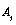to quantify the ability of the j-th plane andis associated with the typical cruise speed of each plane. This variable turns out to be useful in determine the search plan. Through this process we determine the plan and how we should use the searching plane. Our result is good. We only use 8 planes and 1.6 hours to find the debris of AF-477.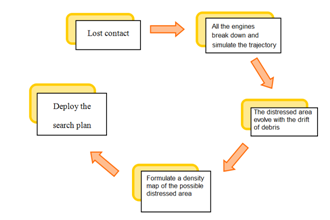Figure 1. The Overall Process of Optimal Search and Rescue Model.

2. Search and Rescue Optimization Model Building

2.1. Basic Assumptions and Reasons Behind

In practice, the motion of the pre-distress and drift after the distress are difficult to compute due to the irregular geometry of real-world objects. Thus simplifications must be introduced to make the problem tractable, and with these simplifications errors will also be introduced.

The height and velocity of the plane as well as the scheduled route are known. We simplify the route by considering a line between point A to point B

In the pre-distress stage, when plane is still in the air, we assume the all the engines fail to work after it leaves the radar surveillance.

It is assumed the plane still keeps balance before its crash.

We neglect the case of a hurricane during the flight. The aerodynamics would be too complicated if we want to compute the impact of strong wind.

We know the position and time of last appearance on the screen.

Assume every search plane use up all the fuel when it finishes a searching task.

Neglect the takeoff and landing time for each searching plane.

2.2. Locating the Area of Debris

2.2.1. Pre-distress Period

We say a plane is missing when it disappears from the radar, but generally it may not crash immediately. As no signal is received by the search and rescue team, there will be two scenarios of the pre-distress motion. First is that the plane still functions well for some time, for instance, terrorists hijact the plane. In fact, according to the types of the missing plane, we can calculate the maximum distance where the plane could fly. And we assume the engines stop working at time t1. Since no more information can be known, it is reasonable think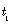has a normal distribution, which means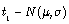, whereand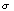can be determined empirically. Besides, the plane can deviate from the scheduled route, so we assume the possible area to be a disc with radius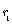.Then we can we get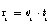, where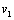means the speed of the plane at this stage.

Secondly, once the all of the engines stop working at time, it is reasonable to believe that the plane will gradually descend if the pilots keep the plane in balance. We simplify the following process similar to a Projectile motion, in which the plane moves along a curved path under the action of gravity and the force resistance of wind. Also we take the lift force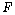into consideration. And we always don't know which direction it will fly, so the possible area is again a disc with radius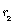. The following graph shows how we analyze it.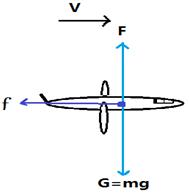Figure 2. The force diagram of the airplane after t1.

Thus we get the following differential equations.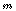is the mass of the plane. There are three forces influence the plane: gravity, air resistance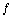and lift power. By Newton's second law, we have: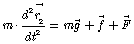Along the x axis, there is only one force, the air resistance, which is impacting on the plane. It is quite normal that we assume the air resistance is correlated with the speed of the object. So it can be modeled as a function of the speed which is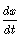here. Therefore, we get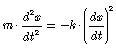Alone the y-axis, there are 2 forces, gravity and lift force by air. They are in the opposite direction: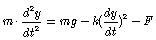Set the initial position where the plane begins to fall as: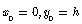, hence by solving the ordinary differential equation, we get the result:Therefore we can formulate the trajectory for Boeing 777-200ER as follows: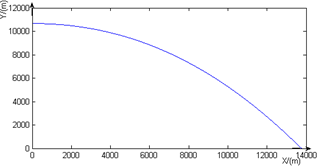Figure 3. The trajectory path of Boeing 777-200ER starting from t1.

The plane will move within a disc with radius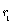before. And then at time, the plane could be at any point in that disc, say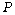. After that it will again fall into a disc centered atwith radius. Thus we can easily check that the possible area is again a disc with radius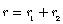.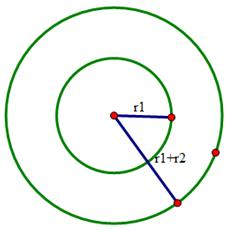Figure 4. The simulated possible region for the distress plane.

2.2.2. Post-Distress Period

After the plane crashed into water, it might break down. But generally different parts of the debris would not scattered around greatly because the time before the searching team to arrive should not be too long. So we ignore the distance between different parts of the debris and consider the wrecked plane as a single point. As for the leeway (We follow the definition by Hodgins and Mark (1995)  that "The leeway is the drift associated with wind forces on the exposed above-water part of the object"), there are existed way to compute it . The approach used by the US Coast Guard consider the downwind and crosswind component of the leeway, and use a linear regression model  to simulate the trajectory of drift. Then the simulator produce probability density maps of the object’s location using a particle filter where each particle represents a possible path for the search object.

To take a simpler approach, we basically take only one unknown into account, the current direction. Because the above water part of debris will not be of great height, which suggests area of the surface on which wind force can act is negligible. Therefore we can ignore the effect of wind here in our model. Furthermore, the model assumes the current to be regular and always move in the same direction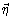. The direction ofis the current direction, while the absolute value of it is the speed of current. Therefore we consider the effect of current as a move of the possible searching area in pre-distress stage without changing the area's size. The following figure shows the case: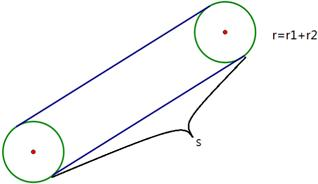Figure 5. The change of the region with the movement of ocean current.

Let the current speed be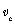, and the search and rescue team arrive at time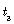. The displacement is: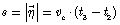2.2.3. Relationship Between the Types of the Lost Plane and the Search Area

As we have shown above, several stages of calculation will involve in the special properties of the downed plane. First of all, when we compute the trajectory, we must take the mass of plane into account. Besides, the lift force of the air also depends on the surface area of the plane. Take MH370 as an example. The Boeing 777-200ER has a gross weight of 152845 kg. Using this set of data, the computation result foris 14.142 km. And the searching area is 628.3 km2. So we can see the types of planes do influence the searching range and it is necessary to consider the properties of the planes before we deploy searching.

Table 1. The parameter of the planes for MH370 and AF477.

 Types Typical cruise height (km) Gross weight (kg) Typical cruise speed(km/h) Possible area (km2) Boeing777 10.668 152845 233 628.3 Airbus330 10.668 180000 240 724

2.3. The Optimal Search Plan Updated by Bayes Method

The process has three main components as follows: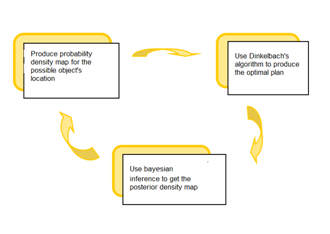Figure 6. Three main components of the plan.

Table 2. The sweep widths of fixed-wing aircraft.

 Search Object Altitude 500ft Altitude 1000ft Visibility (km) Visibility (km) 2 5 10 20 30 >40 2 5 10 20 30 >40 Person in water 0.0 0.1 0.1 0.1 0.1 0.1 0.0 0.1 0.1 0.1 0.1 0.1 Raft 1 persons 0.3 0.7 0.9 1.2 1.4 1.4 0.3 0.7 0.9 1.2 1.4 1.4 Raft 4 persons 0.4 1.0 1.3 1.8 2.0 2.2 0.3 1.0 1.3 1.8 2.1 2.3 Raft 6 persons 0.4 1.1 1.5 2.2 2.5 2.8 0.4 1.1 1.6 2.2 2.6 2.8 Raft 8 persons 0.4 1.2 1.6 2.3 2.7 2.9 0.4 1.2 1.7 2.4 2.8 3.0 Raft 10 persons 0.4 1.2 1.7 2.5 2.9 3.2 0.4 1.3 1.8 2.6 3.0 3.3 Raft 15 persons 0.5 1.3 1.9 2.7 3.3 3.6 0.4 1.4 2.0 2.8 3.4 3.7 Raft 20 persons 0.5 1.5 2.1 3.2 3.8 4.2 0.4 1.5 2.2 3.2 3.9 4.3 Raft 25 persons 0.5 1.6 2.3 3.4 4.1 4.6 0.4 1.6 2.3 3.5 4.2 4.7

2.3.1. Evaluation of Searching Ability of Different Types of Planes

In our paper, we mainly give a plan for search and rescue only using planes. So it is significant to evaluate the capability (denoted as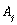) of specific search planes. We consider it in the following way.

Because the search work requires the pilot to sweep by eyes using telescope or infrared detector, the plane cannot go at its maximum speed. Under the pressure of time, the plane could not go too slow as well. So it is most reasonable to let the plane fly at its typical cruise speed. That explains whyis determined by the cruise speed, and the sweep search width. After searching the data, we find that the preferable height adopted by most search and rescue team are 500 feet or 1000 feet. Here is a table we found on Adoption of Amendments to The International Aeronautical and Maritime Search and Rescue Manual.

Therefore we define: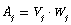Basic information of 8 different types planes which are frequently used in search and rescue is as the following:

Table 3. Parameters related to searching ability of different planes.

 Types Cruise speed(km/h) Maximum range (km) Maximum speed(km/h) Duration T(h) Aj C-130 602 7876 ---- 13.1 120.4 P-3C 608 8945 761 12.3 121.6 P-8A 815 8000 902 13 163 MH-60G 133 2400 361 18 26.6 HH-60 250 1920 355 13.08 50 AW109 220.75 3856 285 13.08 44.15 EC145 246 2720 268 11.2 49.2 EC135 254 2540 281 10 50.8

In the following part, we will useto determine the optimal plan to assign planes in searching task.

2.3.2. Quick Scheduled Route Search

The aim of the search at this stage is to quickly go through the route of the downed plane. The reason is that we cannot get any signal from the downed plane, so the plane is most probable to stay around its scheduled route. Normally, at this stage the pilot scan the objects in the surface of ocean by eyes. Thus we require the plane to be low in altitude. Denote the center of the searching area to be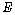, and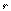is the radius of the searching area. The maximum distance of the sweep by eyes is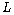. The following figure is a sketch of the route should be adopted by searching planes. This practice has been used as a ritual process at the beginning of search.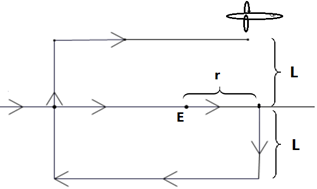Figure 7. Route adopted by searching planes.

2.3.3. Optimal Plan Based on Dinkelbach's Algorithm

It is likely that the quick air route search has no findings. Meanwhile the area we plan to search tends to be larger due to the drift of debris. Here again we assume there is an equal probability for the debris to drift alone every direction. In order to minimize the overlap of different planes searching area, we draw tangent lines to the original area thus a square is formed around it. Then, we divide the area into several smaller areas and assign a searching plane to each area at one time.Figure 8. Divide the area and assign them to different searching plane.

After a plane been assigned to a specific area, we need to determine how to carry out searching task in an efficient manner. Generally, planes will take rather more time to turn its head around than normal cruise. For this reason, we want the plane to fly along the long edge of the given area rather than along the short edge. We can save a lot of time by reducing the number of times turning around.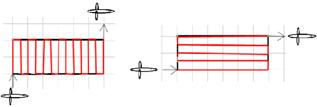Figure 9. The path should be adopted by the searching plane.

Then we need to consider the next stage's search. Here we use 0-1 fractional program for the rescue, which means we use a parameter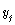to determine whether to use the j-th plane or not. If we use it, the value is 1, else the value is 0. Here we assume there are N planes come to search in total, and the area waiting to be searched is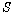. Also, we denote the total time of the search to be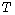, which means at this time we cover the whole area using different planes. Considering there are different types of plane come to rescue, for the j-th plane, we denote the maximum cruise time to be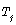, and we always assume every plane runs up the timefor each task. Furthermore we neglect the time for the planes to fuel up again, as well as the time used when taking off and landing. And there is no time interval between two successive task.  Thus the j-th plane would have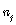tasks, where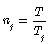Furthermore, it is easy for us to calculate the time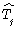, that the j-th plane takes flying from the base to the searching area. And in the total time, we denote the j-th planes' total time spending on the search as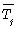. Then we can have the following equation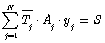And then considering the cost, it is reasonable to assume that we can only assign a total number of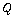tasks to the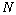planes. In that way, we can formulate a "0-1 Fractional Programming Problems" as follows:According to different value of parameters in specific case, which can be found in the model evaluation part, we can use Dinkelbach algorithm to get a solution for. This set of solution contains the information whether to use a plane or not. It is easy to handle and we can make a quick decision on the search and rescue plan. Therefore we can assign tasks to different planes in an optimal way.

2.3.4. Initial Probability Density Map of the Object's Location

Since the area may evolve with current and wind, the size of the area which contains debris may become larger and larger. So it would be helpful to locate a rather small area of the highest probability. After that we give priority to the target area when we are doing search and rescue work.

The evolution will be affected by so many unpredictable factors, such as the continuous change of wind and current, the shape of the debris etc. It is hard and complicated to choose a dominant factor or formulate a model to describe the trajectory without a large amount of data. A simple and natural assumption is that the point of distress follows a 2-dimensional (bivariate) normal distribution, with mean 0 and variance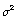.Thereafter the value ofcan be approximated by previous data. If the total area is still too large, we can go even further to reduce it by using '3-'rule, which contends that with probability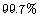the plane will fall into an area within a radius of 3 standard deviations from the central point. The following figure is the probability density map we get using '3-'rule.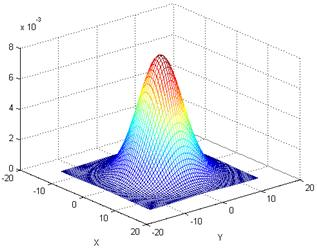Figure 10. The initial probability distribution for searching.

2.3.5. Updating the Density Map by Bayesian Inference

We generally don't want to repeat the search in an area again and again. Since it is a probabilistic problem to decide which area we should choose first, we might think after a search with no findings, the probability of that area would decrease. Following this intuition, we update the object's density function using Bayesian inference.

Suppose a grid square has a probability p of containing the wreck and that the probability of successfully detecting the wreck if it is there is q. If the square is searched and no wreck is found, then, by Bayes' theorem, the revised probability of the wreck being in the square is given byHere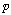is given by the initial distribution in the last section. And we assumeis associated with,which means the greater the plane's capability is, the less chance that it will miss the debris given that the debris does exists in that area.

2.4. Model Application on Air France Flight AF477

Our model yields a nice result in the prediction of the object's area. As mentioned in the above section, we obtain the radius of search area is 15.181km. And the Probability density function for the object location is as follows:Figure 11. Probability density map of the object's location for AF477.

Compare to the actual site where most of the debris has been found, our model fits the reality well by covering the debris in searching area. The following picture shows the debris site and our predicting area.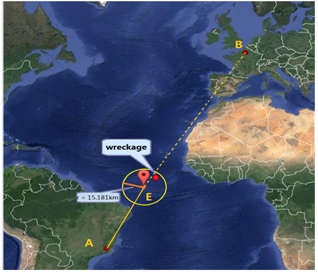Figure 12. Actual site and predicting search area.

As for the searching plan, it is assumed that the searching team has one plane of every type mentioned earlier when evaluating the planes' searching capability, including helicopters (MH-60G,AW109), Maritime patrol aircraft(P3-C),and military transport aircraft (C-130), etc.

Using Dinkelbach's algorithm, the searching plan developed in this case works efficiently. It only takes 1.64 hour till the first debris has been sighted using 8 given planes, and 1.91 hour using 7 planes.

2.5. Model Application on Malasian Flight MH370

The Malaysia Airlines Flight 370 was a scheduled international passenger flight that disappeared on Saturday, 8 March 2014, while flying from Kuala Lumpur International Airport near Kuala Lumpur, Malaysia to Beijing Capital International Airport in Beijing, China. The aircraft, a Boeing 777-200ER, was carrying 12 Malaysian crew members and 227 passengers from 15 nations.

Firstly, according to the height and typical cruise speed of Boeing 777-200ER, we calculate the trajectory of MH370: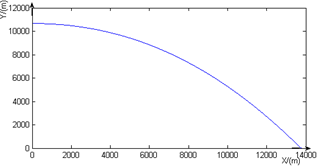Figure 13. The trajectory path of Boeing 777-200ER starting from t1.

Table 4. The result of 0-1 fractional programming.

 Q(No.of planes) Y1 Y2 Y3 Y4 Y5 Y6 Y7 Y8 T(hour) 1 0 0 1 0 0 0 0 0 4.75 2 0 1 1 0 0 0 0 0 2.72 3 1 1 1 0 0 0 0 0 1.96 4 1 1 1 0 1 0 0 0 1.85 5 1 1 1 0 1 1 0 0 1.78 6 1 1 1 0 1 1 1 0 1.71 7 1 1 1 0 1 1 1 1 1.66 8 1 1 1 1 1 1 1 1 1.64

Secondly, we solve the 0-1 fractional programming. Here again we are given the same eight types of planes as given in the search plan for AF477. In addition, it is assumed that the number of planes used is left to the team's discretion, so that the strategy of using the planes can be adjusted if there is any mechanical failure of any one of these planes. Table 4 shows the result of the 0-1 fractional programming.

References

1. International Civil Aviation Organization (ICAO), International Maritime Organization (2006). Adoption of Amendments to the International Aeronautical and Maritime Search and Rescue (IAMSAR) Manual (MSC/Circ.999,1044.180,1124), 190-199.
2. Russell A, Quigley J, van der Meer R. (Apr. 2006). Modeling the reliability of search and rescue operations within the UK through Bayesian belief networks. Proceedings of the First International Conference on Availability, Reliability and Security, 810-816.
3. Weiguang Zhang, Jianchu Kang, Hesong Li. (2007). A global optimization algorithms based on cloud model. Journal of Beijing University of Aeronautics and Astronautics, 33(4), 486-490.
4. Hannah Bast, Susan Hert. (2000). The Area Partitioning Problem. Proceedings of 12th Canadian Conference on Computational Geometry, 163-172.
5. Susan Hert, Brad Richards. (2002). Multiple-Robot Motion Planning Parallel Processing and Geometry. Lecture Notes in Computer Science, Volume 2238, 195-215.
6. David Adjiashvili, David Peleg. (2008). Equal-Area Locus-Based Convex Polygon Decomposition. Lecture Notes in Computer Science, Volume 5058, Structural Information and Communication Complexity, 141-155.
7. J Mark Keil, Tzvetalin S Vassilev. (2006). Algorithms for optimal area triangulations of a convex polygon. Computational Geometry: Theory and Applications, 35(3):173-187.
8. Hert Susan, Lumelsky Valdimir. (1998). Polygon Area Decomposition for Multi-robot Workspace Division. International Journal of Computational Geometry and Applications (S0218-1959), 8(4):437-466.
9. Lawrence D. Stone and Thomas M. Kratzke, Metron Inc, John R. Frost. (2011). Search Modeling and Optimization in USCG’s Search and Rescue Optimal Planning System (SAROPS). U.S. Coast Guard, France.
10. Oyvind Breivik, Arthur A. Allen.(2008). An Operational Search and Rescue Model for the Norwegian Sea and the North Sea. J Marine Syst, 69(1-2), 99-113.

 Contents 1. 1.1. 1.2. 1.3. 2. 2.1. 2.2. 2.3. 2.4. 2.5.
Article ToolsAbstractPDF(2991K)